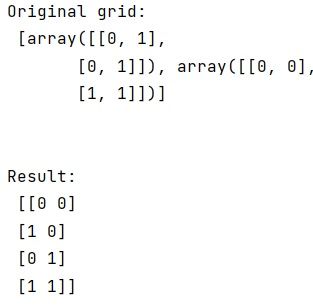# How to convert the output of meshgrid() to the corresponding array of points?

Learn, how can we convert output of meshgrid() to corresponding array of points? By Pranit Sharma Last updated : March 29, 2023

Suppose that we want to create a list of points that would correspond to a grid. So, if I want to create a grid of the region from (0, 0) to (1, 1), it would contain the points (0, 0), (0, 1), (1, 0), and (1, 0).

Now, the grid can be constructed using numpy.meshgrid(). We need to know how to convert this grid into the corresponding set of array points?

## Converting the output of meshgrid() to an array of points

To converting the output of meshgrid() to an array of points, we will simply reshape the grid and find the transpose of the grid which will simply result in the corresponding array points.

Let us understand with the help of an example,

## Python code to convert output of meshgrid() to corresponding array of points

```# Import numpy
import numpy as np

# Creating a grid
gr = np.meshgrid([0,1],[0,1])

# Display Original grid
print("Original grid:\n",gr,"\n\n")

# Creating array points
res = np.array(gr).reshape(2, -1).T

# Display result
print("Result:\n",res)
```

### Output